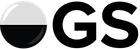Miai Counting when Tally == 0

The miai value of a position is calculated by taking the point swings from each player playing first in the position and summing their absolute values, and then dividing the sum by the sum of the absolute values of the respective differences in how many moves were played by each player in the respective hypothetical playouts, as I understand it. In a normal game I’d count the above position as 4 points miai because (2 + 2)/(1) = 4, under the shortcut that sente has a Tally of 1 and gote of 2. But how is the Tally here not 0? Shouldn’t that be undefined? Is it saying that it is impossible to assign a value to a double-sente play? Is the actual answer sensical in the complex plane, and only appears nonsensical because we’re only calculating the real part? Maybe the magnitude of the vector from the origin to a way of calculating Tally which outputs a complex number would give a sensible answer?

This is significant because I’m trying to figure out how to count the value of plays in Thue-Morse Go, and I need something more robust than a binary gote-sente distinction.

I know absolutelly nothing on this, having skipped over the below link. But have a look at it. but as i remember the resident expert would be someone like @BHydden i think.

Check this out.

1 Like

Maybe This?

• floor(x...): a variadic function which takes in 2 or more real numbers and returns the value of the smallest.
• abs(x): a function which takes in 1 real number and returns the absolute value of that number.

• BScore: the local score (B = positive, W = negative) if Black plays first.
• WScore: the local score (B = positive, W = negative) if White plays first.
• BStones: the number of stones Black plays locally in the sequence.
• WStones: the number of stones White plays locally in the sequence.
• BTally: abs(BStones - WStones) when Black plays first.
• WTally: abs(BStones - WStones) when White plays first.

• Swing: abs(BScore - WScore)
• Tally: floor(BTally, WTally) + 1

• MiaiValue = Swing/Tally

Conclusion

This gives (if I understand correctly) the same results as Miai Counting when playing orthogo, and seems to give reasonable results in TMG (Thue Morse Go). Does anyone have any better answers to the OP?